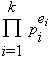# Fundamental Theorem of Arithmetic

If a number n is not prime, then we often seek to factor it into primes (that is, write it as a product of primes). Does such a factorization always exist? And is this factorization unique? About 300 BC Euclid answered yes in his Elements. Today we word his answer as follows:

The Fundamental Theorem of Arithmetic
Every positive integer greater than one can be expressed uniquely as a product of primes, apart from the rearrangement of terms.

The canonical (or standard ) form of the factorization is to write n =where the primes pi satisfy p1 < p2 < ... < pk and the exponents are positive integers. For example, 60 = 22.31.51. We can reword the Fundamental Theorem this way: the canonical factorization of an integer greater than one is unique.

This theorem (and indeed any theorem labeled "fundamental") should not be taken too lightly. There are many number systems in which factorizations are not unique. For example, suppose we only had the even integers (add and multiply as usual), and call a number "e-prime" if it is not the product of two other even numbers. Then the e-primes are 2, 6, 10, 14, 18, . . . Notice that 36 has two different factorizations: 62 = 2.18. (See if you can create some other examples.)

So what is it that makes the fundamental theorem hold? Basically two properties: first, that every integer can be written as a product of primes (this is a simple consequence of the well ordering principle); and second, if a prime p divides ab, then p divides a or b (this is sometimes used as the definition of prime, see the entry prime number).

Finally, the reader might enjoy seeing how Euclid worded this theorem. In proposition 14 of Book IX he wrote:

If a number be the least that is measured by prime numbers, it will not be measured by any other prime except those originally measuring it.

Here Euclid uses "measure" to mean what we call "divide," and he is thinking of it in a very concrete geometric sense: 3 measures 12, because 4 line segments of length 3 has the same length as one line segment of length 12.